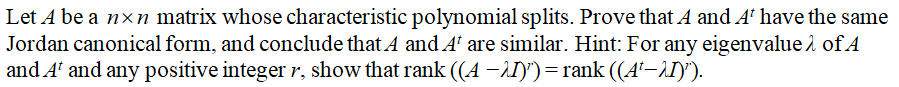# Let A be an n×n matrix whose characteristic polynomial splits. Prove that A and At have the same Jordan canonical form, and conclude that A and At are similar. Hint: For any eigenvalue λ of A and At and any positive integer r, show that rank((A −λI)r) = rank((At−λI)r).

Question
102 views

Let A be an n×n matrix whose characteristic polynomial splits. Prove that A and At have the same Jordan canonical form, and conclude that A and At are similar. Hint: For any eigenvalue λ of A and At and any positive integer r, show that rank((A −λI)r) = rank((At−λI)r).

check_circle

Step 1...

### Want to see the full answer?

See Solution

#### Want to see this answer and more?

Solutions are written by subject experts who are available 24/7. Questions are typically answered within 1 hour.*

See Solution
*Response times may vary by subject and question.
Tagged in

### Math